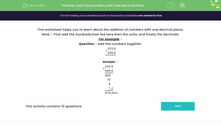# Add Two Numbers with One Decimal Place

In this worksheet, students add together two numbers with one decimal place.Key stage:  KS 2

Curriculum topic:   Number: Fractions and Decimals

Curriculum subtopic:   Solve Fraction and Decimal Problems

Difficulty level:#### Worksheet Overview

In this activity, we'll be practising how to add two numbers with one decimal place.

Top Tip - We always start first by adding the hundreds, then the tens, then the units, and finally the decimals.

Let's have a look at an example of how we can write this down...

Question - Can you add the following numbers together?

 + 3 3 3 . 6 6 4 5 . 6

So, how can we do this?  Remember we start with the hundreds column first.

300 + 600 = 900

30 + 40 = 70

3 + 5 = 8

0.6 + 0.6 = 1.2

900 + 70 + 8 + 1.2 = 979.2

Tip: Laying work out in columns helps stop us from making mistakes when we add.

 + 3 3 3 . 6 6 4 5 . 6 9 0 0 7 0 8 1 . 2 9 7 9 . 2

Let's get started!

### What is EdPlace?

We're your National Curriculum aligned online education content provider helping each child succeed in English, maths and science from year 1 to GCSE. With an EdPlace account you’ll be able to track and measure progress, helping each child achieve their best. We build confidence and attainment by personalising each child’s learning at a level that suits them.

Get started